‘கேட் ஆர்கிடெக்சருக்கு’ வரவேற்கிறோம். ‘গেট আৰ্কিটেকচাৰ’লৈ আপোনাক স্বাগতম। ‘ગેટ આર્કિટેક્ચર’માં આપનું સ્વાગત છે ‘गेट आर्किटेक्चर’ में आपका स्वागत है। ‘ಗೇಟ್ ಆರ್ಕಿಟೆಕ್ಚರ್’ ಗೆ ಸುಸ್ವಾಗತ. ഗേറ്റ് ആർക്കിടെക്ചറിലേക്ക് സ്വാഗതം. ‘गेट आर्किटेक्चर’ मध्ये आपले स्वागत आहे. ‘గేట్ ఆర్కిటెక్చర్’కి స్వాగతం. ‘گیٹ آرکیٹیکچر’ میں خوش آمدید۔

The GATE exam is very near, on 4th Feb. 2023. Each day counts. Download our eBooks in 5 minutes and start reading. Buy now to download for ₹35 each, the Question Bank with ESSENTIAL NOTES. The best thing is, you can start reading from your mobile phone itself even if you are waiting for, on a bus stop!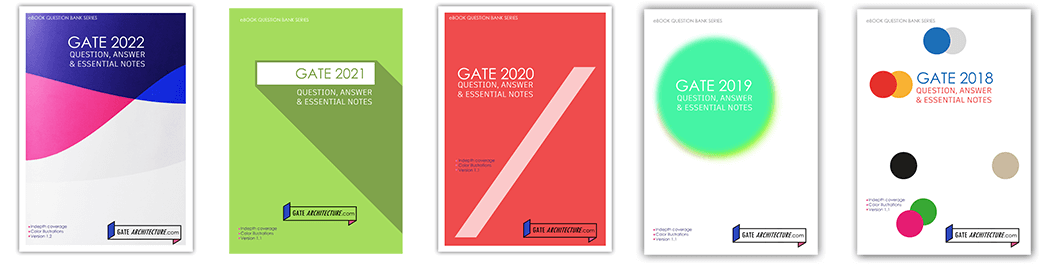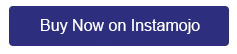Followings are poll results about GATE 2022 AR.

1. Was GATE AR 2022 difficult when compared to GATE AR 2021?

Total Voters: 282Loading ...

2. Do you welcome the NEW question paper pattern with optional Sections B1 & B2 based on updated syllabus?

Total Voters: 234Loading ...

3. Which optional Section did you attempt?

• B1 (Architecture) (77%, 189 Votes)
• B2 (Planning) (23%, 58 Votes)

Total Voters: 247Loading ...

4. What do you think the expected cut-off would be?

• 31 to 33 (32%, 64 Votes)
• 34 to 37 (30%, 61 Votes)
• 38 to 41 (23%, 46 Votes)
• 42 to 45 (16%, 32 Votes)

Total Voters: 203Loading ...

The cut-off (general) is 37.3. One question of 2 marks in aptitude section is awarded ‘marks to all’ candidates!

5. About the composition of the question paper.

• At least 50% of the question paper was weighted towards numericals. (58%, 106 Votes)
• It was well balanced between numerical and non-numerical type questions. (42%, 77 Votes)

Total Voters: 183Loading ...

6. How many aptitude based questions did you solve?

• 6 to 8 (45%, 86 Votes)
• All 10 questions (38%, 73 Votes)
• 1 to 5 (16%, 31 Votes)

Total Voters: 190Loading ...

7. Did you have enough time to answer all the questions?

• It was just enough. (64%, 116 Votes)
• Extra 15 minutes would have done good. (23%, 41 Votes)
• Could not attemp all questions. (14%, 25 Votes)

Total Voters: 182Loading ...

8. How many silly mistakes/blunder did you make in the exam?

• More than 2 mistakes. (80%, 128 Votes)
• 2 mistakes. (10%, 16 Votes)
• 1 mistake. (6%, 10 Votes)
• Questions were straight forward. No scope for mistake. (4%, 7 Votes)

Total Voters: 161Loading ...

9. How many questions were asked directly or indirectly from last 5 years of question paper?

• 1 to 3 questions (40%, 61 Votes)
• 4 to 5 questions (12%, 18 Votes)
• More than 5 questions (10%, 15 Votes)

Total Voters: 153Loading ...

• It was tuff and time consuming. (70%, 104 Votes)
• It was scoring. (12%, 18 Votes)

Total Voters: 148Loading ...

11. How many questions would you go for review?

• More than 2 questions (51%, 59 Votes)
• 2 questions (13%, 15 Votes)
• 1 question (7%, 8 Votes)

Total Voters: 115Loading ...

• OK but needs a fair amount of improvements. (27%, 30 Votes)

Total Voters: 111Loading ...

Our curated GATE Question Bank is also good for various exams meant for job hunt in Architecture & Planning. It contains ESSENTIAL NOTES so as to provide extra reading on the subject related to a particular question so that you would be able to answer the question related to a particular subject.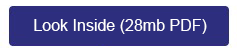GATE 2022 AR QUESTION PAPER

Congratulations to all who have qualified GATE. Those who could not get through, all the best for next endeavour.

General Aptitude Section, 15 Marks (1×5 + 2×5)

Question 1: Grammar (1 mark)

After playing _________ hours of tennis, I am feeling _________ tired to walk back.

(A) too / too

(B) too / two

(C) two / two

(D) two / too

Explanation: I am feeling too tired to walk back. ⇒ I am so much tired that I can not walk back.

Question 2: Quantitative Aptitude (1 mark)

The average of the monthly salaries of M, N and S is ₹ 4000. The average of the monthly salaries of N, S and P is ₹ 5000. The monthly salary of P is ₹ 6000.
What is the monthly salary of M as a percentage of the monthly salary of P?

(A) 50%

(B) 75%

(C) 100%

(D) 125 %

Explanation: (M + N + S)/3 = 4000 ⇒ M + N + S = 12000 …equation (a)

(N + S + P)/3 = 5000 ⇒ N + S + P = 15000 ⇒ N + S + 6000 = 15000 ⇒ N + S = 9000 …equation (b)

Putting the value of N + S from eq. (b) in eq. (a):

M + 9000 = 12000 ⇒ M = 3000

Requirement of the question: (M/P)*100% = (3000/6000)*100% = 50% Answer

Question 3: Quantitative Aptitude (1 mark)

A person travelled 80 km in 6 hours. If the person travelled the first part with a uniform speed of 10 kmph and the remaining part with a uniform speed of 18 kmph.
What percentage of the total distance is travelled at a uniform speed  of 10 kmph?

(A) 28.25

(B) 37.25

(C) 43.75

(D) 50.00

Explanation: Let, first part of the distance of 80 km covered be x in time t.

So, t = x/10  …equation (a)

Therefore, for second part of journey:

6-t = (80-x)/18 ⇒ t = 6 – (80-x)/18 …equation (b)

Equating both equations:

x/10 = 6 – (80-x)/18 ⇒ x = 35

Requirement of the question: (x/80)*100% = (35/80)*100% = 43.75 Answer

Question 4: Analytical Aptitude (1 mark)

Four girls P, Q, R and S are studying languages in a University. P is learning French and Dutch. Q is learning Chinese and Japanese. R is learning Spanish and French. S is learning Dutch and Japanese.
Given that: French is easier than Dutch; Chinese is harder than Japanese; Dutch is easier than Japanese, and Spanish is easier than French.
Based on the above information, which girl is learning the most difficult pair of languages?

(A) P

(B) Q

(C) R

(D) S

Question 5: Spatial Aptitude (1 mark)

A block with a trapezoidal cross-section is placed over a block with rectangular cross-section as shown below.

Which one of the following is the correct drawing of the view of the 3D object as viewed in the direction indicated by an arrow in the above figure?

(A)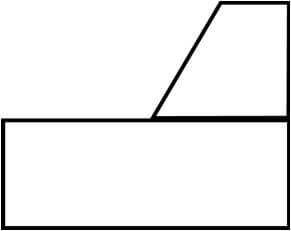(B)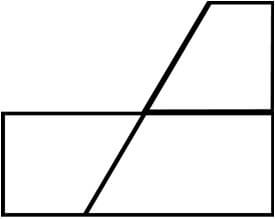(C)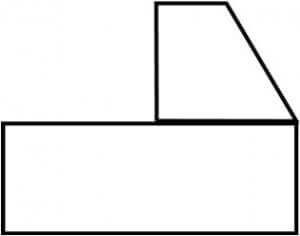(D)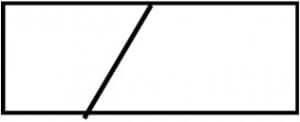Question 6: Verbal Aptitude (Comprehension), 2 marks

Humans are naturally compassionate and honest. In a study using strategically placed wallets that appear “lost”, it was found that wallets with money are more likely to be returned than wallets without money. Similarly, wallets that had a key and money are more likely to be returned than wallets with the same amount of money alone. This suggests that the primary reason for this behavior is compassion and empathy.
Which one of the following is the CORRECT logical inference based on the information in the above passage?

(A) Wallets with a key are more likely to be returned because people do not care about money

(B) Wallets with a key are more likely to be returned because people relate to suffering of others

(C) Wallets used in experiments are more likely to be returned than wallets that are really lost

(D) Money is always more important than keys

Explanation: Option (A) is wrong because, the paragraph never states explicitly that “people do not care about money”. However, the paragraph states comparatively that “wallets that had a key and money are more likely to be returned than wallets with the same amount of money alone”

Option (C) is wrong because the person that returned wallets never knew that it was an experiment. This is because the wallets were strategically placed that appeared to be “lost”.

Option (D) is wrong because the reverse might be correct to a some extent. Keys would be more important than money because “humans are naturally compassionate”. Human might think that money could be earned but lost keys would make it harder to find a solution. Read the paragraph by replacing keys with either PAN card or DL.

Question 7: Quantitative Aptitude (2 marks)

A rhombus is formed by joining the midpoints of the sides of a unit square.  What is the diameter of the largest circle that can be inscribed within the rhombus?

(A) 1/√2    (B) 1/2√2

(C) √2       (D) 2√2

Explanation, a rhombus will be a square when it is formed by joining the midpoints of the sides of a square. Also, the diameter of the circle will be same as the side of the rhombus (square). So, the question is essentially asking the length of the hypotenuse of a triangle with base 1/2 and height 1/2. Therefore, length = √[(1/2)2 + (1/2)2] = 1/√2 Answer

Question 8: Quantitative Aptitude (2 marks)

An equilateral triangle, a square and a circle have equal areas.  What is the ratio of the perimeters of the equilateral triangle to square to circle?

(A) 3√3 ∶ 2 ∶√𝜋

(B) √(3√3) : 2 ∶√𝜋

(C) √(3√3) : 4 ∶2√𝜋

(C) √(3√3) : 2 ∶2√𝜋

Explanation: Let, side of a triangle, square and circle be a, b and c respectively.

As per question, 1/2(a)*(√3/2a) = b*b = π*c2 = k (assume)

Therefore, a2 = 4k/√3, b2 = k and c2 = k/π

Requirement of question,

perimeter of triangle : perimeter of square : perimeter of circle

⇒ 3a : 4b : 2πc

⇒ 9a2 : 16b2 : 4π2c

⇒ 9(4k/√3) :16k : 4π2(k/π)

⇒ 9(4/√3) :16 : 4π2(/π)

⇒ 3√3 : 4 : π

Squarerooting all:

Question 9: Analytical Aptitude (Reasoning), 2 marks

Given below are three conclusions drawn based on the following three statements.
Statement 1: All teachers are professors.
Statement 2: No professor is a male.
Statement 3: Some males are engineers.
Conclusion I: No engineer is a professor.
Conclusion II: Some engineers are professors.
Conclusion III: No male is a teacher.
Which one of the following options can be logically inferred?

(A) Only conclusion III is correct

(B) Only conclusion I and conclusion II are correct

(C) Only conclusion II and conclusion III are correct

(D) Only conclusion I and conclusion III are correct

Question 10: Quantitative Aptitude (2 marks)

In a 12-hour clock that runs correctly, how many times do the second, minute, and hour hands of the clock coincide, in a 12-hour duration from 3 PM in a day to 3 AM the next day?

(A) 11     (B) 12   (C) 144    (D) 2

Initially, option (A) was the answer as per first released answer key by official GATE. Later in the final answer key (after challenge/review), marks was awarded to all candidates. In GATE 2021 AR, four questions were given ‘marks to all’. Is the question is framed in such a way that at least one or two questions are put intentionally to kill time of a examinee and later awarded ‘marks to all’ or framing a question is so difficult that at least one question is awarded ‘marks to all’?

Part A Section: Architecture based questions. Compulsory for all

Question 11: Solar Architecture (1 mark)

The concentric circles in a sun-path diagram represent ___________.

(A) Altitude angle      (B) Azimuth angle

(C) Day of the year    (D) Hour of the day

Explanation:

Reference: Lechner, N. (2001), Heating, Cooling, Lighting: design methods for architects, second edition, USA: John Wiley & Sons

Question 12: National Housing Policy (1 mark)

The operational guidelines on Credit Linked Subsidy Scheme for Economically Weaker Sections (EWS), January 2017, by the erstwhile Ministry of Housing & Urban Poverty Alleviation, Government of India, defines EWS households as those having an annual income up to ___________ (in Indian Rupees).

(A) 2,00,000    (B) 2,50,000    (C) 3,00,000   (D) 3,50,000

EWS: Economically Weaker Section households are defined as households having an annual income up to Rs. 3,00,000 (Rupees Three Lakhs).

EWS House: An all weather single unit or a unit in a multi-storeyed super structure having carpet area of upto 30 sq. m. with adequate basic civic services and infrastructure services like toilet, water, electricity etc.

Carpet Area: Area enclosed within the walls, actual area to lay the carpet. This area does not include the thickness of the inner walls

LIG: Low Income Group households are defined as households having an annual income between Rs.3,00,001 (Rupees Three Lakhs One) up to Rs.6,00,000 (Rupees Six Lakhs).

Question 13: Architectural Graphics (1 mark)

Which of the following is a Vector Graphics Software?

(A) Inkscape (B) Odeon (C) Adobe Dreamweaver (D) DesignBuilder

Question 14: Structure (1 mark)

The main cable of a suspension bridge supports the deck with hangars. These hangars are equidistant along the length of the bridge and represent a uniformly distributed load. Assuming the cable to be weightless as compared to the applied loading, the best approximation of the shape that the cable takes for this loading is a ___________.

(A) Catenary curve (B) Circular arc  (C) Parabolic curve (D) Hyperbolic curve

What a coincidence! The banner photograph of this website depicts a suspension bridge.

Question 15: Hierarchy of roads (1 mark)

Arrange the following road types in descending order of accessibility.

(Q) Expressway
(S) Local Street

(A) Q-P-R-S

(B) S-R-P-Q

(C) S-P-R-Q

(D) P-Q-S-R

Explanation: Local street is most accessible.

Question 16: Design Principle (1 mark)

The following two-dimensional visual composition represents ___________.

(A) Interlocking

(B) Intersecting

(C) Interlacing

(D) Interpenetrating

Question 17: Visual composition in 2D (1 mark)

The Golden Ratio refers to ___________.

(A) 1:√2  (B) 2:(1 +√5)  (C) 1:1 (D) 16:9

Question 18: Architectural graphics (1 mark)

Hogarth’s Line of Beauty is a ___________.

(A) Horizontal straight line

(B) Zigzag line

(C) Vertical straight line

(D) Serpentine line

Question 19: Natural and man-made ecosystem, Current affair (1 mark)

Which of the following sites were added to Ramsar List in the year 2020?

(A) Ashtamudi Wetland

(B) Asan Conservation Reserve

(C) Chilika Lake

(D) Lonar Lake

Question 20: Climate responsive design, (1 mark)

Which of the following help(s) in keeping direct solar radiation out of the building?

(C) Malquf (D) Chajja

Question 21: Professional practice and ethics (1 mark)

As per the Handbook of Professional Documents 2015, Council of Architecture, India, architects are liable ___________.

(A) If the building is used for any other purpose than the one for which it was designed

(B) If any unauthorised changes or illegal modifications are made by the owner(s)/occupant(s)

(C) If the client suffers damage/loss due to lack of proper professional service

(D) If the architect fails to attain the standard of care as prescribed by law

Question 22: Sustainable development, (1 mark)

As per the United Nations Transforming our world: The 2030 agenda for sustainable development, 2015, which of the following Sustainable Development Goals (SDGs) directly address water related issues?

(A) SDG – 1

(B) SDG – 2

(C) SDG – 6

(D) SDG – 14

Explanation: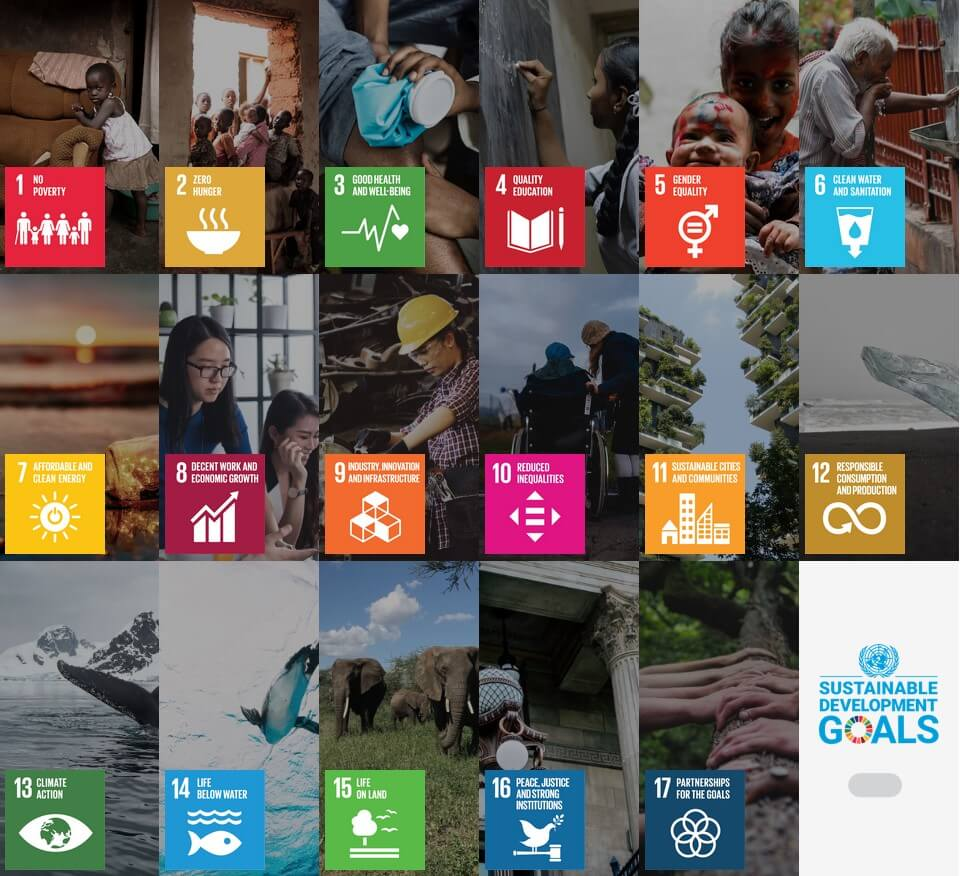The 17 Sustainable Development Goals (SDGs), which are an urgent call for action by all countries – developed and developing – in a global partnership.

Reference: https://sdgs.un.org/goals

Question 23: Structure (1 mark)

For a masonry section, the line of action of force shifts to incorporate the effects of lateral forces and induced moments. Consider a masonry section of width 600 mm. Assuming a zero tensile stress capacity and a linear stress-strain response for the entire domain of loading, the minimum value of eccentricity at which the section will crack (in mm, rounded off to one decimal place) is ___________.

Notes: This is NOT a numerical question. So, you need not to solve anything.

This question seems to be from the code “IS: 1905; 1987 (1998) Indian Standard specification for Code of Practice for Structural Use of Unreinforced Masonry”. The code states that “when the span is more than 30 times the thickness of wall, the point of application of the load shall be considered to be displaced from the centre of bearing towards the span of the floor to an extent of one-sixth the bearing wall width.” In a special case, the eccentricity of loading lies between 1/3 and 1/2 of the thickness of the member.

Therefore, the answer should be in one sixth of 600 mm = 100 Answer

Question 24: Thermal comfort in built environments (from B1 syllabus), 1 mark

The maximum and minimum indoor dry bulb temperature of a room are 38°C and 34°C, respectively. If the corresponding outdoor maximum and minimum dry bulb temperature are 42°C and 30°C, respectively, then the thermal damping of the room (in percentage, rounded off to two decimal places) is ___________.

Solution: Thermal Damping, D = [(To – Ti)/To]*100% =[(12 – 4)/12 ]*100% = 66.67 % Answer

Where, To = Outside temperature range

Ti = Inside temperature range

Reference: Page 5 of IS: 3792 – 1978: Indian Standard Guide for heat insulation of non-industrial building.

Question 25: Site planning (1 mark)

A building site measures 96 sq.cm on a scale of 1:12500. The actual area it represents (in hectare, in integer) is ___________.

Solution: Let the building site on the map be of square shape. Its area given is 96 sq.cm. So, its one side will be √(96 sq.cm) = 9.8 cm.

Therefore, in real, one side of the building site will be 9.8 cm x 12500 = 9.8 x 125 m = 1225 m.

Hence, the area of the building site will be 1225 m x 1225 m = 1500625 sq.m = 1500625 / 100×100 ha = 150 hectare Answer

(100 m x 100 m = 1 hectare)

Question 26: Transportation (1 mark)

An off-street car parking lot contains a total of 75 bays. If the parking lot was used by 687 cars over a period of 12 hours, the average parking turn-over of the parking lot (in vehicles per hour per bay, rounded off to two decimal places) is ___________.

Solution: Parking turnover = [(No. of vehicles/parking duration)] / No. of parking bays = [(687cars/12hours)]/75bays = 0.76 cars per hour per bay Answer

Question 27: Planning and Design of storm water drainage system (1 mark)

The hydraulic radius of the following rectangular open drainage section (in mm, rounded off to two decimal places) is ___________.

Solution: Hydraulic radius = A/P, where A is the cross sectional area of flow and P is its wetted perimeter.

A = 600mm x250 mm

P = 250mm + 600mm + 250m = 1100mm

Therefore, Hydraulic radius = A/P = (600 x 250)sq.mm / 1100mm = 136.36 mm Answer

Question 28: Methods of solid waste management (disposal), 1mark

A town with 0.45 million population sends its entire organic waste to a composting site on a daily basis through a truck of 15 ton carrying capacity. Assume total waste generated per capita per day is 0.21 kg and 40% of the total waste is organic waste.  The minimum number of weekly round trips required by the truck (in integer) will be ___________.

Solution:  In 1 day, organic waste generated and disposed same day = 40% of (0.21 kg * 450000) = 37800 kg

No. of truck trips required to dispose all waste generated same day = 37800 kg / 15000 kg = 2.52 ⇒ 3 trips

Therefore, total trips in a week = 21 trips Answer

Question 29: Construction and Management (2 marks)

The correct sequence of the following Construction Project Development stages, as per the National Building Code of India 2016 is ___________.

(P) Resource Planning
(Q) Project Inception
(R) Commissioning and Handing over
(S) Tendering
(T) Site Survey and Soil Investigation
(U) Selection of Construction Methodology

(A) P-Q-R-T-U-S

(B) T-Q-R-U-S-P

(C) Q-T-U-P-S-R

(D) Q-T-P-S-U-R

Question 30: Mixed syllabus (2 marks)

Match the aspects in Group I with the corresponding items in Group II.

(A) P-4, Q-5, R-2, S-3   (B) P-5, Q-1, R-4, S-2

(C) P-2, Q-4, R-5, S-1   (D) P-2, Q-5, R-4, S-3

Question 31: Match the States in Group I with the corresponding Vernacular Building Typologies in Group II

(A) P-4, Q-5, R-3, S-2   (B) P-5, Q-1, R-2, S-4

(C) P-5, Q-3, R-1, S-4   (D) P-4, Q-3, R-1, S-2

Question 32: Match the examples in Group I with their corresponding typologies in Group II.

(A) P-2, Q-1, R-4, S-5   (B) P-4, Q-2, R-5, S-3

(C) P-3, Q-1, R-2, S-5   (D) P-3, Q-5, R-1, S-4

Question 33: Match the Place(s)/Event(s) in Group I with the corresponding Heritage Significance/Characteristics in Group II.

(A) P-1, Q-4, R-3, S-2   (B) P-3, Q-4, R-5, S-1

(C) P-2, Q-3, R-4, S-1   (D) P-3, Q-2, R-5, S-4

Question 34: Match the Urban Design Concepts in Group I with their corresponding Proponents in Group II.

(A) P-1, Q-2, R-3, S-4   (B) P-4, Q-1, R-2, S-3

(C) P-4, Q-3, R-5, S-1   (D) P-5, Q-4, R-2, S-3

Question 35: In the following sketch, P, Q, R, and S refer to elements of an urban space. Identify P, Q, R, S.

(A) P-Path, Q-Vista, R- Edge, S-Landmark

(B) P-Vista, Q-Edge, R- Landmark, S-Path

(C) P-Landmark, Q-Vista, R- Path, S-Edge

(D) P-Landmark, Q-Edge, R- Path, S-Vista

Question 36: As per the URDPFI Guidelines 2015, match the type of Health Care Facilities in Group I to the corresponding population served per unit in Group II.

(A) P-1, Q-2, R-3, S-4   (B) P-3, Q-1, R-5, S-4

(C) P-4, Q-3, R-5, S-2   (D) P-5, Q-1, R-2, S-3

Question 37: Match the plan forms in Group I with their corresponding project names in Group II.

(A) P-3, Q-5, R-4, S-2 (B) P-4, Q-2, R-1, S-5

(C) P-1, Q-2, R-3, S-4 (D) P-3, Q-5, R-1, S-2

Question 38: Match the Biosphere reserves in India in Group I with their corresponding locations in Group II.

(A) P-2, Q-1, R-4, S-3 (B) P-2, Q-3, R-1, S-5

(C) P-3, Q-1, R-4, S-5 (D) P-4, Q-5, R-1, S-3

Question 39: In traditional Persian context, qanat system refers to

(A) An underground water-way, tunnelled and channelled

(B) A system where water is raised by a series of scoops fixed to a moving belt stretched between two wheels

(C) A method of conducting water from a source-well rather than raising it

(D) A system where water is conducted from enclosure to enclosure by straightforward gravity fall

Question 40: Which of the following is/are classified as the Principles of Universal Design?

(A) Perceptible Information (B) Tolerance for Error

(C) Occult Balance              (D) Simple and Intuitive Use

Explanation: Universal design is a framework for creating living and working environments and products that serve the broadest possible range of people in the broadest possible range of conditions without requiring unique or distinct design. Followings are 7 Principles of Universal Design:

1: Equitable Use

2: Flexibility in Use

3: Simple and Intuitive Use

4: Perceptible Information

5: Tolerance for Error

6: Low Physical Effort

7: Size and Space for Approach and Use

Question 41: As per the URDPFI Guidelines 2015, which of the following Organoleptic and Physical parameters comply with the acceptable limit requirements of drinking water quality?

(A) Colour: Maximum 5 Hazen units

(B) Turbidity: Maximum 1 NTU

(C) pH Value: Minimum 10

(D) Total Dissolved Solids: Maximum 500 mg/l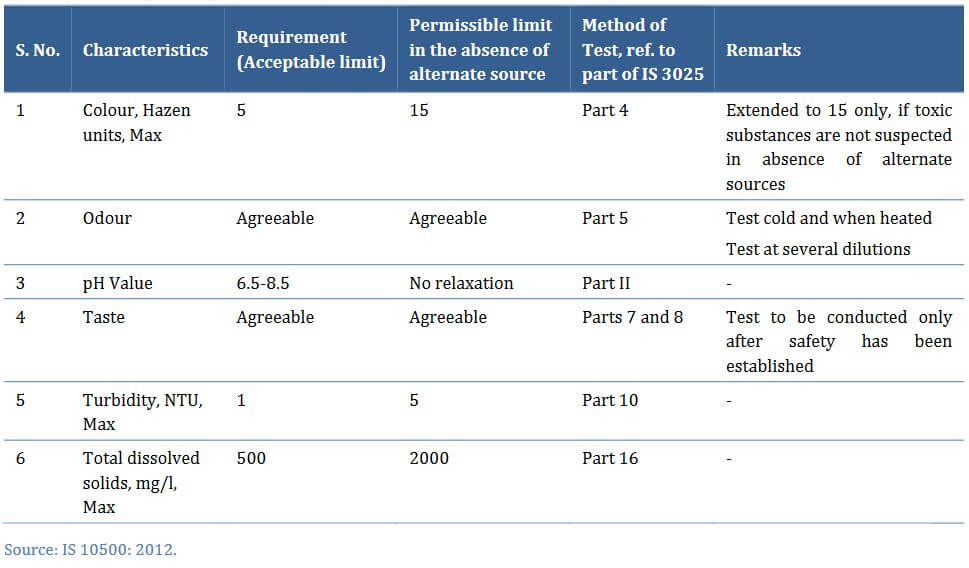Table 8.29 : Organoleptic and Physical Parameters of Drinking Water, Source: URDPFI Guidelines Volume 2, page 319

Question 42: In an ideal air-conditioning cycle shown below, which of the following statement(s) is/are true in the segments P, Q, R, S?

(A) P: Vapour at low pressure

(B) Q: Vapour at low pressure

(C) R: Liquid at high pressure

(D) S: Liquid-Vapour mixture at low pressure

Question 43: Which of the following is/are the characteristic(s) of a Mughal Garden?

(A) Symmetrical and geometrical                    (B) Fountain and channelled water

(C) Winding road and untrimmed vegetation (D) Vista with terminal building

Question 44: As per the Central Pollution Control Board’s National Air Quality Index (AQI) of India 2014, which of the following statement(s) is/are true?

(A) AQI is computed considering 8-hourly value of CO

(B) AQI is computed considering 2-hourly value of PM2.5

(C) AQI considers the O3 concentrations

(D) AQI considers the CO2 concentrations

Question 45: The decadal population data of a city are given in the following Table. The domestic water consumption of the city is estimated to be 175 litres per capita per day in the year 2041. Considering 2011 population as the base year and using arithmetic growth method of population forecasting, the daily domestic water demand of the city in the year 2041 (in million litres per day, rounded off to two decimal places) will be ___________.

Year: 1981 > 1991 > 2001 > 2011

Population: 1,80,750 > 1,95,850 > 2,15,300 > 2,45,450

Explanation: In every decade, population changes. There are three changes in the question. We need to find the average of these changes and then considering it as base, population is forcasted using airthmetic growth.

Question 46: The activity duration, early start, early finish, late start, and late finish (in weeks) for activities P, Q, R, and S are shown in the following figure. The interfering float of activity R (in weeks, in integer) is ___________.

Total float: It is the amount of time that the completion time of an activity can be delayed without affecting project completion time. (LS – ES or LF -EF)
Free float: It is the amount of time that the activity completion time can be delayed without affecting the earliest start time of the immediate successor activities in the network. (ES of successor – EF of current)
Interfering float: (Fin)
• Maximum amount of time by which an activity can be delayed without delaying the project but will cause delay to the Early Start of some following activity
• It is the difference between total float and free float. It is thus equal to the head event slack.
Fin = Ft- Ff = 12 – 6 = 6 Answer
Where, Ft is Total Float = 12, Ff is Free float = 6
Independent Float: Amount by which an activity can be delayed without delaying the project; even if all predecessors are at late Finish and all Successors are at Early Start.

Question 47: A 230 mm thick brick wall of 10 m length and 3 m height is built using a Flemish bond. The size of the bricks used is 230 mm x 112.5 mm x 70 mm. Assuming a mortar thickness of 5 mm, the number of bricks required (in integer) is ___________.

Solution: Let 3 bricks in the pattern given below makes 1 set. Its total length is 352.5 mm.

For 10 meters (10000 mm), no. of sets = 10000/352.5 = 28.37

Therefore, total no. of bricks 28.37 x 3 = 85.11 = 85 bricks (0.11 translates into 0.11 x 352.5 = 39 mm = 4 cm and it can be ignored)

Height of 1 brick layer = 75 mm (including mortar thickness). So, number of layers in 3m = 3000/75 = 40 layers

1 layer requires 85 bricks,

Therefore, 40 layers require 40 x 85 = 3400 bricks Answer

Question 48: The reflected ceiling plan and section of a reinforced cement concrete roof are shown in the following Figure. All the beams are 300 mm wide, 600 mm deep (including 150 mm slab) equidistantly placed center to center. Assuming 1% of concrete volume is occupied by reinforcement bars, the volume of concrete (in cubic meters, rounded off to two decimal places) is ___________.

Solution: Volume without 9 depressions = 10 m x 10 m x 0.6 = 60 m3

Length of side of a BIG square (adding all 9 small squares) =  10m – 4 x 0.3 = 8.8 m

Volume of 9 depressions (BIG square) = 8.8m x 8.8m x 0.45m = 34.85m3

So, Volume of reinforced concrete roof = 60 – 34.85 = 25.15 m3

99% of 25.15 m3 = 24.90 Answer

Question 49: The following graph represents the income distribution among the population of a country. The Gini Coefficient of the country (rounded off to three decimal places) is ___________.

Solution:

Part B1 Architectural Section: Optional (Attempt either B1 or B2)

Question 50 (B1): Which of the following processes is used for surface treatment of metals?

(A) Soldering (B) Thermoplating (C) Extrusion (D) Riveting

Question 51 (B1): Among the following monuments of ancient Greece, the only Octastyle Peripteral temple with eight towering Doric columns lining both east and west facades is ___________.

(A) Temple of Athena (B) Temple of Apollo (C) The Parthenon (D) Temple of Horus

Question 52 (B1): An Ultrasonic Pulse Velocity (UPV) test was done on a hardened concrete element using a direct transmission method as per IS 516 (Part 5/Section 1): 2018. The distance between the transducer and receiver was 600 mm. The time taken for the induced wave to travel this distance is measured as 0.18 milliseconds. Based on the following Table, the concrete quality grading is ___________.

(A) Excellent (B) Good (C) Doubtful (D) Poor

Solution: 1000 millisecond = 1 second

Speed = Distance / Time = 600 mm / 0.18 millisecond = 0.6m / (0.18/1000s) = (0.6/1000 km) / (0.18/1000s) = 3.33 km/s ⇒ Doubtful

Question 53 (B1): Which of the following is/are example(s) of Tomb Architecture of Ancient Egypt?

(A) Step Pyramid of Zoser, Sakkara (B) Great Temple of Abu-Simbel (C) Temple of Khons, Karnak (D) Mastabas of Gizeh

Question 54 (B1): If Aluminium : Anodisation :: Glazing : X, which of the following choices  represent X?

(A) Hard coating (B) External cement plastering (C) Tempering (D) Free-standing vertical greening

Question 55 (B1): A blackbody radiant heating panel of 5 m2 surface area at 35 °C surface temperature is located 1 m away from a 1 m2 surface at 20 °C. The Stefan-Boltzmann constant is 5.6703×10−8W m−2K−4. The rate of radiant heat emission by the radiant heating panel (in W, rounded off to two decimal places) is ___________.

Solution: The rate of heat transfer by emitted radiation is determined by the Stefan-Boltzmann law of radiation:

where σ is the Stefan-Boltzmann constant, A is the surface area of the object, and T is its absolute temperature in Kelvin. The symbol e stands for the emissivity of the object, which is a measure of how well it radiates. An ideal jet-black (or black body) radiator has = 1, whereas a perfect reflector has = 0. Real objects fall between these two values. Take, for example, tungsten light bulb filaments which have an e of about 0.5, and carbon black (a material used in printer toner), which has the (greatest known) emissivity of about 0.99. (Source: https://courses.lumenlearning.com/physics/chapter/14-7-radiation/)

The net radiation will be proportional to the temperature difference but area of the radiating body only irrespective of the distance.

So, =

Note, T = 273 + 35 = 308 (°C should be converted into Kelvin as 0°C is marked as 273 Kelvin)

Question 56 (B1): A hypothetical truss comprising of weightless members is shown in the following Figure. Assuming tension to be positive and compression to be negative, the value of force in member TU (in kN, rounded off to one decimal place) is ___________.

Solution:

Question 57 (B1): Match the illustrations of Arch Types in Group I with their corresponding names in Group II

(A) P-2, Q-3, R-1, S-4 (B) P-3, Q-1, R-2, S-5

(C) P-3, Q-2, R-5, S-4 (D) P-5, Q-4, R-3, S-1

Question 58 (B1): Match the architectural projects in Group I with their corresponding architects in Group II.

(A) P-4, Q-5, R-2, S-3 (B) P-4, Q-1, R-5, S-2

(C) P-2, Q-4, R-5, S-1 (D) P-3, Q-5, R-1, S-2

Question 59 (B1): Match the structural joining systems in Group I with the corresponding materials for which they are commonly used in Group II.

(A) P-4, Q-1, R-2, S-5 (B) P-3, Q-5, R-1, S-2

(C) P-2, Q-3, R-5, S-1 (D) P-4, Q-1, R-5, S-3

Question 60 (B1): Match the Instruments in Group I with the corresponding climate parameters in Group II.

(A) P-3, Q-5, R-1, S-2 (B) P-3, Q-4, R-5, S-2

(C) P-5, Q-3, R-2, S-4 (D) P-1, Q-2, R-3, S-5

Question 61 (B1): In traditional Indian temple architecture, which of the following statement(s) is/are true?
(A) Jagamohana refers to a dancing hall

(B) Gopuram refers to an entrance tower

(C) Char-chala refers to a roof composed of four triangular segments

(D) Vimana refers to the structure over the Garbhagriha

Question 62 (B1): Which of the following factors impact Daylight Autonomy of a built space?

(A) Orientation of building (B) Glare caused by daylight

(C) Latitude and longitude of building location (D) Fenestration size

Question 63 (B1): For the beam shown in the following Figure, assuming a sagging moment (generating tensile stresses at the bottom fibre) as positive and a hogging moment (generating tensile stresses at the top fibre) as negative, the bending moment (in kN.m, rounded off to one decimal place) at section X-X is ___________.

Solution:

Question 64 (B1): The acoustical absorption of a wall panel in each octave band is tabulated below.  The Noise Reduction Coefficient of the wall panel (rounded off to two decimal places) is ___________.

Solution: Noise reduction coefficient, or NRC, is a rating used to measure how effective a material is at absorbing sound. NRC ratings are calculated by taking the arithmetic averages of a material’s sound absorption coefficients at 250, 500, 1000, and 2000 Hz and then rounding it to the neared 0.05.

NRC = (0.5 + 0.5 + 0.7 + 0.8) / 4 = 2.5/4 = 0.62 Answer

Question 65 (B1): A room is maintained at a wet bulb temperature 25°C, globe temperature of 30°C, and air velocity of 0.5 m/s. The decrease in Tropical Summer Index when the air velocity is increased to 3 m/s (in °C, rounded off to two decimal places) is ___________.

Solution: Tropical Summer Index is the temperature of calm air at 50% relative humidity which imparts the same thermal sensation as the given environment. TSI evaluates indoor thermal comfort level.
TSI = 0.745DB + 0.308WB – 2.060V1/2
+ 0.8
Where, DB – Dry Bulb Temperature, WB – Wet Bulb Temperature, V – Wind Speed (m/s)

As DBT and WBT remains unchanged, calculation becomes easy:

ΔTSI =  – 2.060*31/2 – (– 2.060*0.51/2) = – 2.11 Answer

Part B2 Planning Section: Optional (Attempt either B1 or B2)

Question 66 (B2) 1 mark: Which of the following is the National Electronic Toll Collection System implemented by the National Payment Corporation of India?

(A) e-Pass (B) E-ZPass (C) HashTag (D) FASTag

Question 67 (B2) 1 mark: The shaded area in the following demand-supply graph is known as ___________.

(A) Consumer Surplus (B) Consumer Deficit

(C) Producer Surplus (D) Producer Deficit

Question 68 (B2 1 mark): Identify the following traffic interchange.

(A) Directional (B) Trumpet (C) Clover-Leaf (D) Diamond

Question 69 (B2) 1 mark: Which of the following is/are Value Capture Method(s)?

(A) Building construction fees

(B) Fees for changing agricultural to non-agricultural land use

(C) User charge

Explanation: As the additional value is generated by actions other than landowner’s direct investment, Value Capture is distinct from the user charges or fees that agencies collect for providing services.

Reference: Value Capture Finance Policy Framework, Page no. 10, Ministry of Urban Development, Govt. of India.

Question 70 (B2) 1 mark: Which among the following is/are model(s) of Public-Private Partnership (PPP) used for infrastructure projects?

(A) BOLD (B) BOLT (C) BOOT (D) BPOT

Question 71 (B2) 1 mark: The measured spot speeds (in km/h) of 10 vehicles from a traffic stream are 45, 35, 25, 51, 45, 38, 61, 42, 47, and 49. The Time Mean Speed of the traffic stream (in km/h, rounded off to one decimal place) is ___________.

Solution: It is the simple average of spot speed. So, Time Mean Speed = (Sum of 45, 35, 25, 51, 45, 38, 61, 42, 47, and 49) divided by 10 which is equal to 43.8 Answer

Question 72 (B2) 1 mark: In a township, the price of each house was 25,00,000 (in Indian Rupees) last month.  The number of houses sold in a month (Q in thousands) is sensitive to the price of the house (P in Indian Rupees) and establishes a relationship as  Q = 6685 – 0.00158P. If the price of each house increases by 20% in the current month, then the decrease in sale of the houses (in percentage, rounded off to two decimal places) compared to last month will be ___________.

Solution: Q1/Q2*100% = [{(6685 – 0.00158*1.2*2500000)- (6685 – 0.00158*1*2500000)}/(6685 – 0.00158*1*2500000)]*100% = – 28.89

So, decrease in sales in percentage = 28.89 Answer

Question 73 (B2) 2 marks: Match the models in Group I with their corresponding applications in Group II.

(A) P-2, Q-1, R-5, S-4 (B) P-1, Q-5, R-2, S-3

(C) P-2, Q-3, R-5, S-4 (D) P-5, Q-3, R-4, S-2

Question 74 (B2) 2 marks: Match the proponents in Group I with the corresponding theories in Group II.

(A) P-2, Q-4, R-3, S-5 (B) P-4, Q-2, R-5, S-1

(C) P-5, Q-4, R-2, S-1 (D) P-3, Q-5, R-2, S-4

Question 75 (B2) 2 marks: Match the Artists/Scientists in Group I with their corresponding contributions in Group II.

(A) P-4, Q-3, R-1, S-2 (B) P-4, Q-1, R-5, S-2

(C) P-5, Q-3, R-1, S-4 (D) P-4, Q-3, R-5, S-1

Question 76 (B2) 2 marks: In the conceptual diagram of the city given below, P, Q, R, and S refer to urban patterns. Among the choices given below, the correct association is

(A) P-Satellite town, Q-Urban fringe, R-TOD, S-Central Business District

(B) P-Central Business District, Q-Satellite town, R-TOD, S-Urban fringe

(C) P-Urban fringe, Q-TOD, R-Satellite town, S-Central Business District

(D) P-Satellite town, Q-Central Business District, R-TOD, S-Urban fringe

Question 77 (B2) 2 marks: Which among the following is/are the component(s) of the assimilative carrying capacity of urban environment?

(A) Air (B) Water (C) Economy (D) Soil

Question 78 (B2) 2 marks: In the transportation network given below, P, Q, R, S, T, and U are the nodes and values mentioned on the links denote time in minutes. Which of the following options represent the minimum spanning tree?

(A) PQ, QR, QT, TS, SU (B) PR, QR, RT, TU, SU

(C) PQ, QR, RT, TS, SU (D) PQ, QR, RS, ST, TU

Question 79 (B2) 2 marks: A vehicle count survey (in Passenger Car Unit) is conducted on a mid-block section of a road at regular intervals of 15 minutes from 8:00 AM to 10:00 AM. Based on the data given in Table below, the Peak Hour Factor (rounded off to two decimal places) for the given survey duration is ___________.

Solution: The peak hour factor (PHF) is the hourly volume during the maximum-volume hour of the day divided by the peak 15-minute flow rate within the peak hour; a measure of traffic demand fluctuations within the peak hour.

In the given question, we need to select four continuous reading that has combined maximum value. The time slot (8:45 to 9:45) yields maximum value. So, only this slot would be considered! The peak hour volume is just the sum of the volumes of the four 15 minute intervals within the peak hour (315+337 + 405 + 320 = 1377 PCU). The peak 15 minute volume is 405 PCU in this case. The peak hour factor (PHF) is found by dividing the peak hour volume by four times the peak 15 minute volume.

PHF = 1377 /(4 * 405) = 0.85 Answer

Question 80 (B2) 2 marks: A land owner has shown interest in a Town Planning Scheme. Based on the details of the scheme given in the following Table, the estimated Net Benefit to the land owner after land development (in Indian Rupees, in integer) is ___________.

Solution: Initial investment = (500 Sq. m) * (1200 Indian Rupees per Sq.m) = Rs. 6 lakh

Allowed area for development = 60% of 500 Sq. m = 300 Sq. m

Developed land value = (300 Sq. m) * (2800 Indian Rupees per Sq.m) = 8.4 lakh

Profit before tax = 8.4 – 6 = 2.4 lakh

But the land owner has to pay 50% as tax (betterment levy)

So, 50% of 2.4 lakh = 1.2 lakh = 120000 Answer

Question 81 (B2) 2 marks: The year-wise cash flows (in Indian Rupees) of a construction project are given in the following Table. If the annual discount rate for the project is assumed to be 12%, the Net Present Value (in Indian Rupees, rounded off to two decimal places) for the project will be ___________.

Solution:

Explanation:

𝑁𝑃𝑉 =[-5,00,000/ (1+0.12)0] +[0/(1+0.12)1] +[0/(1+.12)2] + [1,30,000/(1+.12)3] +[(1,70,000/(1+0.12)4) +(2,40,000/(1+0.12)5] +[3,30,000/(1+0.12)6 ]= [-5,00,000] + + + [92,531.43] +[1,08,038.07] + [1,36,182.44] + [1,67,188.27] = 3940.1 Answer

11 replies
1.I got AlR 1422 in the general category. What college will I get for M.arch?

2.Praveen says:

Thanks for the solutions. Thank you

3.Praveen says:

And sir for Q.47 brick calculation
If we notice English bond and Double flemish bond 1 brick wall, in both the case brick required as well as mortar required also same. Because in both case same no. of brick and same amt of mortar for a set of 3 if we take. So we can directly calculate Volume of wall /Volume of single brick. Incase of single flemish and double flemish 1.5 brick course the calculation is not like the same.
So,Volume of wall= 10x.23×3= 6.9
Volume of single brick including mortar= .0235 x .1175 x .075= 0.0020709375
No. of bricks req = 6.9/.0020709375= 3331.8= 3332 bricks
Incase if we divide the same by five decimal place= 6.9/ .00207= 3333 bricks
Incase if we divide the same by only four decimal digit= 6.9/.0020 = 3450 bricks

So, answer should include 3332 to 3450 bricks aprox, i think

4.Praveen says:

I have one more doubt Q.46 project management
Interferring float = 1)Total float – free float (or) 2) Late finish – late start
By first method:
Total float= 27-12-3= 12
Free float= 24-12-3= 9 (not 6 i think)
So, Interfering Float= 12-9 = 3 is the answer i think

By method 2) Interfering float = 27-24= 3

Kindly help me whether the above solution is correct or not, because i feel the key answer is wrong for Q.46 & Q.25 also

•Praveen, for Q. 26, the Total Float that you have calculated is right. Alternative solution for calculation of Total Float of R: Late Start of R = 24 weeks and Early Start of R = 12 weeks. So, Total Float of R is 24 -12 = 12 weeks. Free Float = ES of successor – EF of current = ES of successor of R – EF of R = ES of S – EF of R = 21 – 15 = 6 weeks. So, Interfering Float = Total float – Free Float = 12 – 6 = 6. In your calculation of Free Float, you have not considered any thing about of the upcoming activity (successor) and it must be considered because Free Float by definition take cares of upcoming activity.

5.Praveen says:

Aptitude Q.no 9- I diagree on the conclusion, because if some engineers are male as per question. so there are some females. So that female can be an engineer.
Q. 20- keeping direct solar radiation out of building
In this, Mashrabiya avoid direct solar radiation, okay
Badgir, Malquf- wind catchers. But, incase of sunlight, they have solid wall. there is no direct sun enter into the building if we use this Wind towers. As per question this option can also be correct.
Chajja- Chajja will avoid direct sun to some occasion not fully, because western or eastern windows even if it has chajja- direct sun will enter the space. Eg. notice your room which has western window and chajja at the evening.

Q 25.Map 96cm2= .0096m2
1:12500 = 0.0096 x12500= 120sq.m
In hectare= 120/10000 = 0.012 hectare

•Praveen, for question no. 9, your claim ‘female can be an engineer’ is true. But an engineer can be a teacher or professor is NOT defined by the Statements. For question no. 20, Badgir & Malquf are wind catchers. Only slight difference is that one has opening in one direction and other with more than one direction open. These are long towers so as to cool down the wind in the desert. Because of long/tall tower, it may not be used as a daylighting source. If you think your claim is right, you should produce a credible source of information (research paper for example) to prove that either of Badgir and Malquf were used as daylight source while going for a review.

•Q25. In a map, the scale shown is for distance and NOT for area. For example 1:12500 means 1cm on map is equal to 12500cm on site (unit like mm, cm, m etc must be same on either side of the ratio). What you can do as an alternative solution is, you should square both side of the scale ratio. So, 1m : 12500 m > (1m)^2 = (12500m)^2. Therefore, 0.0096m^2 on map will be equal to site area of (0.0096m^2)*(12500*12500) = 15,00,000 m^2 = 150 hectare Answer

6.Praveen Kumar says:

Q.9 conclusion I and III is Correct

7.Praveen says:

Aptitude Q.no 9
Option C, i think

8.